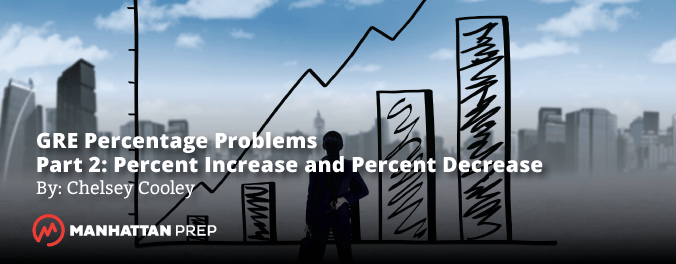GRE Percentage Problems – Part 2: Percent Increase and Percent DecreaseDid you know that you can attend the first session of any of our online or in-person GRE courses absolutely free? We’re not kidding! Check out our upcoming courses here.

If you’ve been following this blog for a while, you’ve probably read our article on how to handle GRE percentage problems. That article teaches you an ultra-simple approach for “percent of” Quant problems — that is, problems like the following:

xy is 20% of z. In terms of y, what percent of x is z?

Unfortunately, not every percentage problem is a “percent of” problem. Some of the toughest problems take things one step further, asking questions like the following:

February’s profits were what percent higher than January’s?

By what percent did the number of students in the school decrease from 2010 to 2011?

Luckily, these problems also have an easy approach. Let’s talk numbers.

It’s finally warming up and getting sunnier here in Seattle, and winter clothes are going on sale. Imagine that a department store originally sold a coat for $100, and it’s just been marked down by 20%. To calculate the sale price, you can do one of two things. 1. You can subtract 20% from 100%, learning that the coat’s current price is 80% of the original price. Then, multiply$100 by 80% to find that the current price is $80. 2. Or, you can find 20% of$100, which is $20. That’s the amount of the discount. Subtract this from$100 to find the current price. Again, it’s $80. So, when you decrease$100 by 20%, you get $80. In other words, 80 is 20% less than 100. Now, imagine the opposite situation. A coat is originally priced at$80. Then, it’s marked up by 20%. Again, you can do one of two things.

1. You can add 20% to 100%, learning that the coat’s current price is 120% of the original price. Multiply $80 by 120% to learn that the current price is$96.
2. Or, you can find 20% of $80, which is$16. That’s the amount of the markup. Add this to $80 to find the current price, which is$96.

In other words, when you increase $80 by 20%, you get$96. You don’t get $100. That’s sort of counterintuitive. It seems like you should be able to decrease a number by 20%, then increase it by 20%, and end up back where you started. The first key to percent increase/decrease problems is to remember that you can’t use ‘increase’ and ‘decrease’ interchangeably. It’s true that 80 is 20% less than 100. But it isn’t true that 100 is 20% greater than 80. If you aren’t careful enough with this, you’ll end up missing problems like the following: 10% more students scored an A on the final exam than scored an A on the midterm. If 110 students scored an A on the final exam, how many students scored an A on the midterm? If you take 110 and decrease it by 10%, you’ll get the wrong answer, 99. Why? Because ‘percent more than’ and ‘percent less than’ aren’t interchangeable. The problem specifies that 110 is 10% more than the number of the students who scored an A on the midterm. You can’t take 10% less than 110 and expect to get the same result. Instead, use a variable, m, for the number of students who scored an A on the midterm. Then, translate the problem into math. Increasing m by 10% corresponds to multiplying m by 1.1. 1.1m = 110 m = 110/1.1 = 100 The correct answer is 100, not 99. Double-check your work by plugging the value back into the problem, and confirming that everything makes sense. If 100 students scored an A on the midterm, then 10% more than that would be 110. That’s the number of students who scored an A on the final exam. It boils down to this: when you write out the math, you have to use exactly what’s written in the problem, including the ‘more than’ or ‘less than’, ‘increase’ or ‘decrease’. The problem above specifies “10% more than”, so no matter what, you’ll have to increase some value in the problem by 10%, rather than decreasing it. Don’t know what that value is? Use a variable to stand in for it. Solve for that variable, and you’ll have your answer. Now, try this one: Pat’s average weekly income from her part-time job was 15% more in September than it was in July. Her average weekly income in July was 10% less than it was in August. If her average weekly income in September was$207, what was her average weekly income in August?

Use variables to stand in for the unknown values. Pat’s weekly income in July was j, and her weekly income in August was a.

The first sentence specifies “15% more than“, so you’ll need to increase j by 15%. That is, 1.15j = 207, so j = 180.

The next sentence specifies “10% less than“, so you’ll need to decrease a by 10%. That is, 0.9a = 180, so a = 180/0.9 = 200. The correct answer is \$200. To review, plug that value back into the problem and confirm that all of the numbers work out correctly.

For even more insight into percentage problems, check out our Fractions, Decimals, and Percents Strategy Guide📝

Want more guidance from our GRE gurus? You can attend the first session of any of our online or in-person GRE courses absolutely free! We’re not kidding. Check out our upcoming courses hereChelsey Cooley is a Manhattan Prep instructor based in Seattle, Washington. Chelsey always followed her heart when it came to her education. Luckily, her heart led her straight to the perfect background for GMAT and GRE teaching: she has undergraduate degrees in mathematics and history, a master’s degree in linguistics, a 790 on the GMAT, and a perfect 170/170 on the GRE. Check out Chelsey’s upcoming GRE prep offerings here.